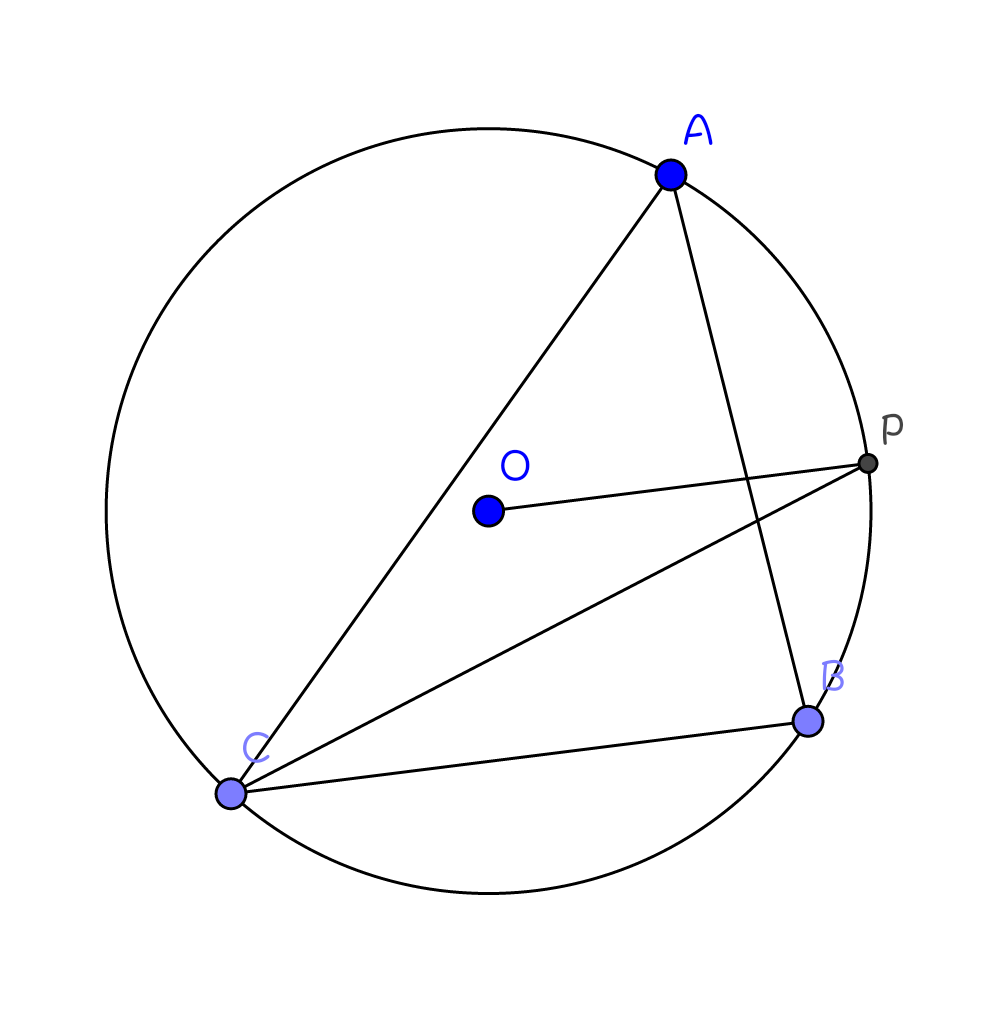# Angle Outlier

Geometry Level 4Circle $O$ is the circumcircle of $\triangle ABC$, and $OP\parallel BC$. If $\angle A=80^\circ$, find $\angle CPO$, in degrees.

×